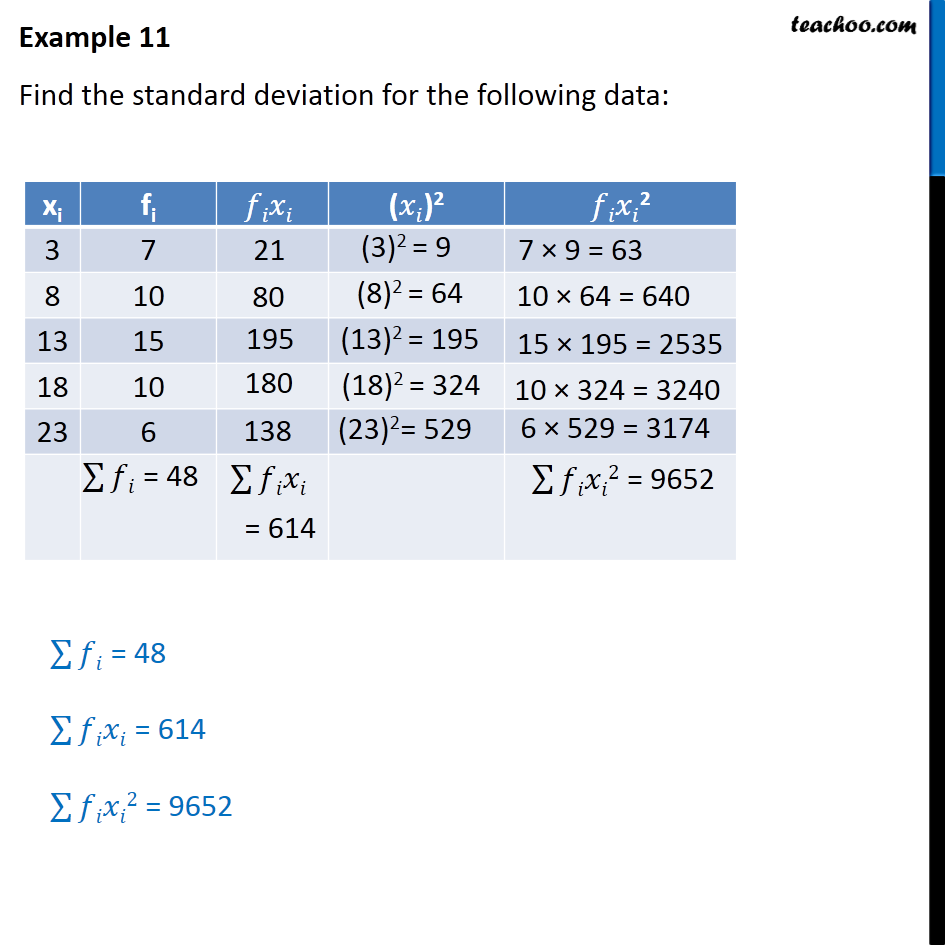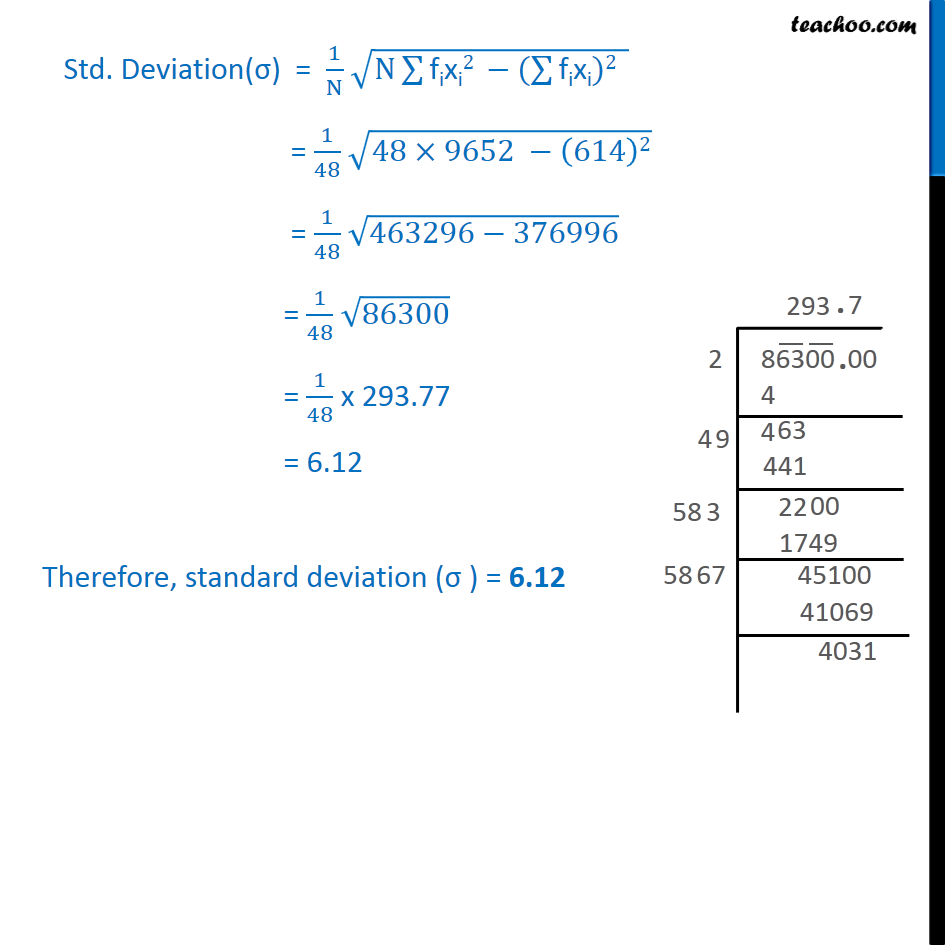Examples

Chapter 13 Class 11 Statistics
Serial order wiseLearn in your speed, with individual attention - Teachoo Maths 1-on-1 Class

### Transcript

Example 11 Find the standard deviation for the following data: Std. Deviation(σ) = 1﷮N﷯ ﷮N ﷮﷮fixi2 −( ﷮﷮fixi)2﷯﷯ ﷯ = 1﷮48﷯ ﷮48×9652 − 614﷯2﷯ = 1﷮48﷯ ﷮463296−376996﷯ = 1﷮48﷯ ﷮86300﷯ = 1﷮48﷯ x 293.77 = 6.12 Therefore, standard deviation (σ ) = 6.12